1. /
2. CBSE
3. /
4. Class 09
5. /
6. Science
7. /
8. NCERT Solutions for Class...

# NCERT Solutions for Class 9 Science Structure of the Atom part 2

NCERT solutions for Science Structure of the Atom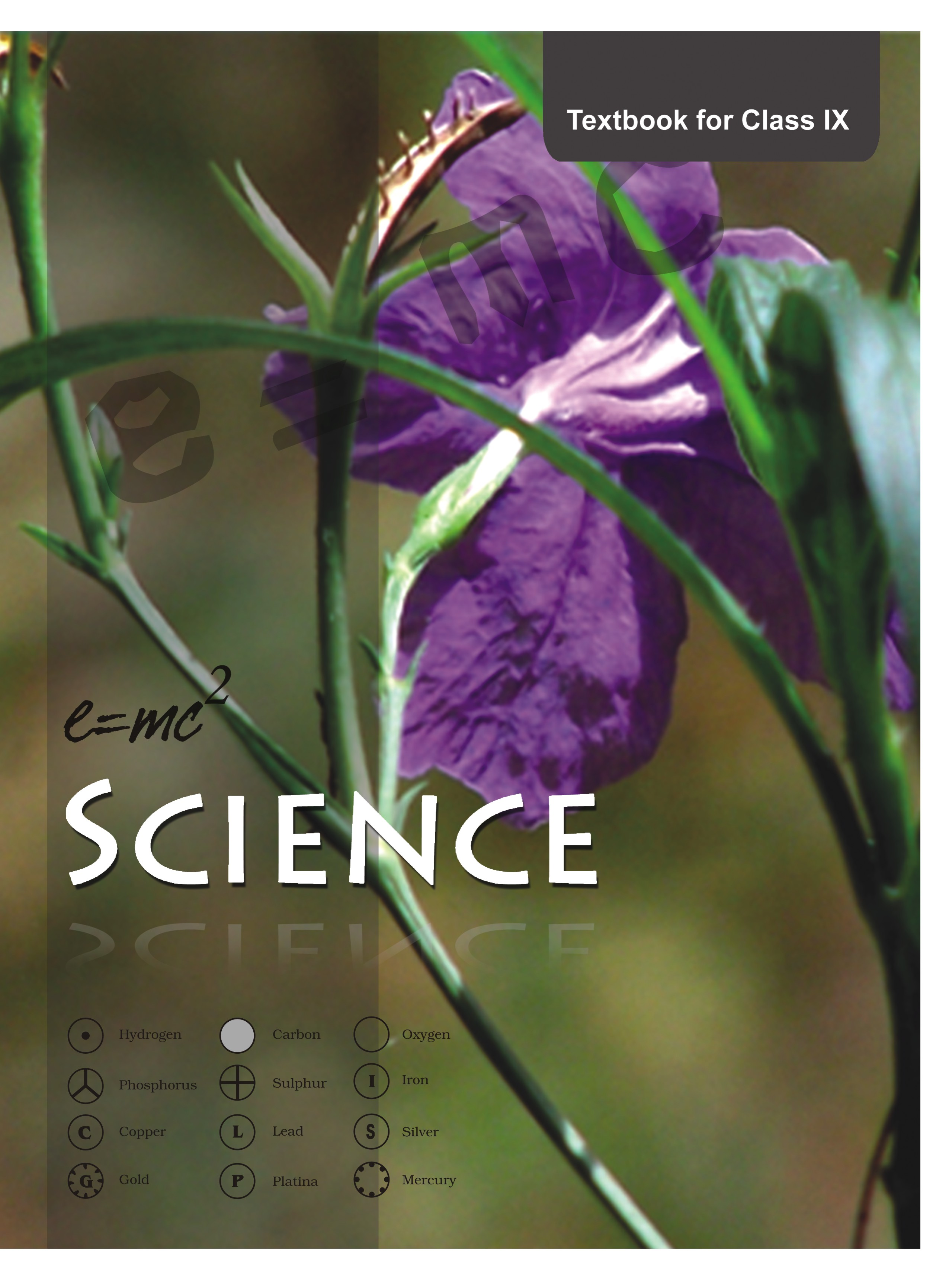## NCERT Class 9 Science Chapter wise Solutions

• 01 –  Matter in Our Surroundings
• 02 – Is Mattter Around us Pure
• 03 – Atoms and Molecules
• 04 – Structure of the Atom
• 05 – The Fundamental Unit of Life
• 06 – Tissues
• 07 – Diversity in Living Organisms
• 08 – Motion
• 09 – Force and Laws of Motion
• 10 – Gravitation
• 11 – Word and Energy
• 12 – Sound
• 13 – Why Do We Fall Ill
• 14 – Natural Resources
• 15 – Improvement in Food Resources

## NCERT Solutions for Class 9 Science Structure of the Atom part 2

###### 1.Compare the properties of electrons, protons and neutrons.

Ans.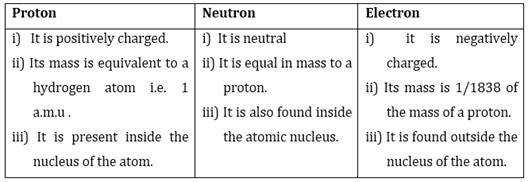###### 2. What are the limitations of J.J. Thomson’s model of the atom?

Ans. Main limitation to the J.J Thomson’s atomic model was that it didn’t explain the arrangement of electrons in an atom.

NCERT Solutions for Class 9 Science Structure of the Atom part 2

###### 3. What are the limitations of Rutherford’s model of the atom?

Ans. Rutherford’s model of atom didn’t explain the stability of atom because as per his model revolving charged electrons while moving through orbit should emit energy and this energy loss will shrink the orbit and ultimately the electron would hit the nucleus and thus atom is unstable but it is not true.

###### 4. Describe Bohr’s model of the atom.

Ans. To remove the drawbacks of Rutherford’s atomic model and to explain structure of atom in detail Neils Bohr in 1912 proposed a model of atom. The special features of Bohr’s model are given below:

i) An electron revolves in the orbit of atom with well-defined energy.

ii) Energy of orbits increases from inner shell to the outer shells i.e. energy for orbit nearest the nucleus is lowest.

iii) If energy is supplied then electron moves from lower orbit ot the higher orbit and if an electron jumps from higher orbit (energy level) to the lower orbit (energy level) then energy is radiated as electromagnetic waves.

iv) Each orbit or shell represents an energy level. Such orbits are represented as K,L,M,N,O……….. and named from centre to outwards.

v) The shell or orbits are associated with certain amount of energy and energy of orbits/shells increases from inward to outwards.eg K<L<M<N<O…………

###### 5. Compare all the proposed models of an atom given in this chapter.

Ans. There are total three atomic models each of which tried to explain the atomic structure in a better way. Following table compares the specific characteristics of the models: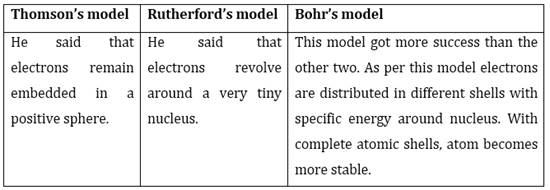NCERT Solutions for Class 9 Science Structure of the Atom part 2

###### 6. Summarise the rules for writing of distribution of electrons in various shells for the first eighteen elements.

Ans. The following rules are followed for writing the number of electrons in different energy levels or shells:

(i) The maximum number of electrons present in a shell is given by the formula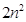, where ‘n’ is the orbit number or energy level index, 1,2,3,… Hence the maximum number of electrons in different shells are as follows:

first orbit or K-shell will be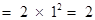,

second orbit or L-shell will be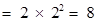,

third orbit or M-shell will be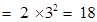,

fourth orbit or N-shell will be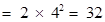, and so on.

(ii) The maximum number of electrons that can be accommodated in the outermost orbit is 8.

(iii) Electrons are not accommodated in a given shell, unless the inner shells are filled. That is, the shells are filled in a step-wise manner.

###### 7. Define valency by taking examples of silicon and oxygen.

Ans. That number of electrons (present in outermost shell) /valence electrons which an atom gives or takes or shares to complete its octet, or acquire stable configuration. Therefore, valency of sulphur = 16= 2,8,6= 2 electrons can be gained easily hence valency is 2.

valency of oxygen = 8 = 2,6 = 2 electrons can be gained easily hence valency is 2.

###### 8. Explain with examples

(i) Atomic number,

(ii) Mass number,

(iii) Isotopes and

iv) Isobars.

Give any two uses of isotopes.

Ans. i) Atomic number = It is the number of protons present inside nucleus of the atom.

It is represented as Z. For eg: for hydrogen Z= 1, because in hydrogen atom the number of protons is 1.

ii) Mass number = It is the total number of protons and neutrons present inside the nucleus of an atom and is represented by A = P + N mass of carbon is 12 u because it has 6 protons and 6 neutrons, 6 u + 6 u = 12 u.

iii) Isotopes = They are atoms of the same element and have same atomic number but different mass number/atomic mass. for example: carbon,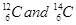iv) Isobars = They are atoms of different elements having same mass number but different atomic number. for example, calcium, atomic number 20, and argon, atomic number 18. The number of electrons in these atoms is different, but the mass number of both these elements is 40. That is, the total number of neutrons is the same in the atoms of this pair of elements.

Two uses of isotopes are as follows:

(i) An isotope of uranium is used as a fuel in nuclear reactors.

(ii) An isotope of cobalt is used in the treatment of cancer.

NCERT Solutions for Class 9 Science Structure of the Atom part 2

###### 9.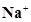has completely filled K and L shells. Explain.

Ans. Na has atomic number 11, so its electronic configuration is = 2,8,1

When it gives away its outermost shell single electron it changes to Na+ =10= 2,8

The above configuration indicates completely filled K,L shells.

###### 10. If bromine atom is available in the form of, say, two isotopes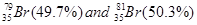. Calculate the average atomic mass of bromine atom.

Ans. The average atomic mass of bromine =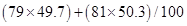= 3926.3 + 4074.3/100

= 8000.6/100

= 80 u

NCERT Solutions for Class 9 Science Structure of the Atom part 2

###### 11. The average atomic mass of a sample of an element X is 16.2 u. What are the percentages of isotopes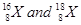in the sample?

Ans. Since average atomic mass =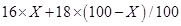16.2=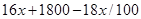1620 =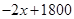2x= 1800 – 1620

x = 180/2 = 90

When 90% is the X-16 sample so for X-18 sample % = 100-90=10%

###### 12. If Z = 3, what would be the valency of the element? Also, name the element.

Ans. When Z = 3, so that element has 3 electrons in its shells distributed as = 2, 1. Its valency is 1 because it can easily give away its outermost single electron and the element is Lithium(Li).

NCERT Solutions for Class 9 Science Structure of the Atom part 2

###### 13. Composition of the nuclei of two atomic species X and Y are given as under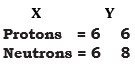Give the mass numbers of X and Y. What is the relation between the two species?

Ans. The mass number of X = 6+6 = 12

The mass number of Y = 6+8 = 14

since their number of protons are same but atomic mass are different so they are isotopes.

###### 14. For the following statements, write T for True and F for False.

(a) J.J. Thomson proposed that the nucleus of an atom contains only nucleons.

(b) A neutron is formed by an electron and a proton combining together. Therefore, it is neutral.

(c) The mass of an electron is about 12000 times that of proton.

(d) An isotope of iodine is used for making tincture iodine, which is used as a medicine.

Ans. (a) false

(b) true

(c) true

(d) false

NCERT Solutions for Class 9 Science Structure of the Atom part 2

###### Put tick (ü) against correct choice and cross (×) against wrong choice in questions 15, 16 and 17

15. Rutherford’s alpha-particle scattering experiment was responsible for the discovery of

(a) Atomic Nucleus

(b) Electron

(c) Proton

(d) Neutron

Ans. (c) proton

NCERT Solutions for Class 9 Science Structure of the Atom part 2

###### 16. Isotopes of an element have

(a) the same physical properties

(b) different chemical properties

(c) different number of neutrons

(d) different atomic numbers.

Ans. (a) the same physical properties

(a) 16

(b) 8

(c) 17

(d) 18

Ans. (b) 8

###### 18. Which one of the following is a correct electronic configuration of sodium?

(a) 2,8

(b) 8,2,1

(c) 2,1,8

(d) 2,8,1.

Ans. (a) 2,8,1

NCERT Solutions for Class 9 Science Structure of the Atom part 2

###### 19. Complete the following table.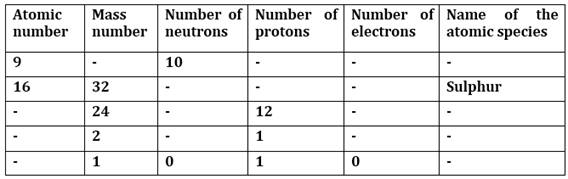Ans.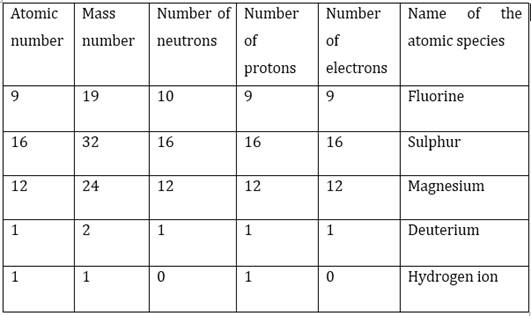## NCERT Solutions for Class 9 Science

NCERT Solutions Class 9 Science PDF (Download) Free from myCBSEguide app and myCBSEguide website. Ncert solution class 9 Science includes text book solutions .NCERT Solutions for CBSE Class 9 Science have total 15 chapters. 9 Science NCERT Solutions in PDF for free Download on our website. Ncert Science class 9 solutions PDF and Science ncert class 9 PDF solutions with latest modifications and as per the latest CBSE syllabus are only available in myCBSEguide.

## CBSE app for Class 9

To download NCERT Solutions for class 9 Science, Computer Science, Home Science,Hindi ,English, Maths Social Science do check myCBSEguide app or website. myCBSEguide provides sample papers with solution, test papers for chapter-wise practice, NCERT solutions, NCERT Exemplar solutions, quick revision notes for ready reference, CBSE guess papers and CBSE important question papers. Sample Paper all are made available through the best app for CBSE students and myCBSEguide website.Test Generator

Create question paper PDF and online tests with your own name & logo in minutes.myCBSEguide

Question Bank, Mock Tests, Exam Papers, NCERT Solutions, Sample Papers, Notes